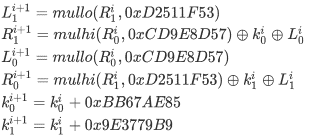ID 772987
Date 12/04/2020
Public

## Philox4x32-10

This is a keyed family of counter-based BRNGs. The state consists of 128-bit integer counter c and two 32-bit keys k0 and k1. The generator has 32-bit integer output obtained in the following way [Salmon2011]:

1. cn = cn-1 + 1

2. wn = f(cn), where f is a function that takes a 128-bit argument and returns a 128-bit number. The returned number is obtained as follows:
1. The argument c is interpreted as four 32-bit numbers, where, put k00 = k0 and k10 = k1.

2. The following recurrence is calculated:Where mulhi(a,b) and mullo(a,b) are high and low 32-bit parts of the a*b product, respectively.

3. Put f(c) =, where N = 10

3. Integer output: r4n+k = wn(k), where wn(k) is the k-th 32-bit integer in quadruple wn, k = 0, 1, 2, 3

4. Real output: un= (int)rn/232+ ½

### Real Implementation (Single and Double Precision)

The output vector is the sequence of the floating-point values u0, u1, ...

### Integer Implementation

The output vector of 32-bit integers r0, r1, ...

### Stream Initialization by Function vslNewStream

Philox4x32-10 generates the stream and initializes it specifying the 32-bit input integer parameter seed. The stream state is a 128-bit number c and a pair of 32-bit integers k0 and k1 initialized in the following way:

1. Assume k0 = seed.
2. Assume the other values are equal to 0, that is k1 = 0 and c = 0.

### Stream Initialization by Function vslNewStreamEx

Philox4x32-10 generates the stream and initializes it specifying the array params[] of n 32-bit integers:

1. If n = 0, assume c = k0 = k1 = 0.
2. If n = 1, assume k = params, c = 0.
3. If n = 2, assume k = params + params*232, c = 0.
4. If n = 3, assume k = params + params*232, c = params.
5. If n = 4, assume k = params + params*232, c = params + params*232.
6. If n = 5, assume k = params + params*232, c = params + params*232+ params*264.
7. If n >= 6, assume k = params + params*232, c = params + params*232+ params*264+ params*296.

### Subsequences Selection Methods

 vslSkipAheadStream Supported vslSkipAheadStreamEx Supported vslLeapfrogStream Not supported

### Generator Period### Empirical Testing Results Summary

Test Name
vsRngUniform
vdRngUniform
viRngUniform
viRngUniformBits
3D Spheres Test OK (20% errors) OK (20% errors) Not applicable OK (20% errors)
Birthday Spacing Test Not applicable Not applicable Not applicable OK (0% errors)
Bitstream Test Not applicable Not applicable Not applicable OK (15% errors)
Rank of 31x31 Binary Matrices Test Not applicable Not applicable Not applicable OK (10% errors)
Rank of 32x32 Binary Matrices Test Not applicable Not applicable Not applicable OK (0% errors)
Rank of 6x8 Binary Matrices Test Not applicable Not applicable Not applicable OK (0% errors)
Counts-the-1’s Test (stream of bits) Not applicable Not applicable Not applicable OK (0% errors)
Counts-the-1’s Test (stream of specific bytes) Not applicable Not applicable Not applicable OK (0% errors)
Craps Test OK (20% errors) OK (20% errors) OK (20% errors) OK (20% errors)
Parking Lot Test OK (0% errors) OK (0% errors) Not applicable OK (0% errors)
2D Self-Avoiding Random Walk Test OK (10% errors) OK (0% errors) Not applicable OK (0% errors)
Notes:
• The tabulated data is obtained using the one-level (threshold) testing technique. The OK result indicates FAIL < 50%. The run fails when p-value falls outside the interval [0.05, 0.95].
• The stream tested is generated by calling the function vslNewStream with seed=7,777,777.